Navigation Panel:(These buttons explained below)Question Corner and Discussion Area

# Understanding Projective Geometry

Asked by Alex Park, Grade 12, Northern Collegiate on September 10, 1996:
Okay, I'm just wondering about the applicability of projective and affine geometries to solving problems dealing with collinearity and concurrence. I'd really like to learn more on the topic, but I'm having trouble finding a book that gives the axioms of them both in a way that I can understand it. As far as I can understand it, there are no such things as parallel lines in projective geometry. How does that work? An explanation would be appreciated.
There are several different ways to think about geometry in general and projective geometry in particular.

## 1. The axiomatic approach

This approach requires no philosophical definition of what a point or a line actually "is", just a list of properties (axioms) that they satisfy. The theorems of geometry are all statements that can be deduced from these properties. In this approach, the theorems of geometry are guaranteed to be true no matter what concept of "point" or "line" is being used and no matter how they are defined, as long as they satisfy the basic axioms.

Euclid wrote down a list of these axioms: five of them (though actually there are some other axioms implicit in Euclid's definitions). He called them postulates. The first four postulates are so self-evident that they clearly ought to be satisfied by anything worthy of the name "geometry". There are different ways of stating them and I don't know which you have seen, so I won't list them, but they basically say things like "for any pair of points, there is a unique line passing through both of them".

However, the fifth postulate isn't quite in the same category. Euclid's version of it was quite complicated; a simpler, equivalent version says that for any line L and a point P not on L, there exists a unique line that is parallel to L (never meets L) and passes through P. For this reason, the fifth postulate is called the parallel postulate.

At first glance it would seem that the parallel postulate ought to be a theorem deducible from the other more basic postulates, rather than something that has to be assumed separately. For centuries mathematicians tried to prove it, but always failed. For example: it follows from Euclid's first four postulates that there is a perpendicular line segment from P to L. Then you can draw a unique line through P that is perpendicular to that line segment. In some sense this is parallel to L because the two angles in the picture below are right angles, but how can one prove from this that this means the lines never intersect? We know they don't in our familiar mental picture of what an infinite flat plane looks like, but is that fact a logical necessity deducible from the other postulates?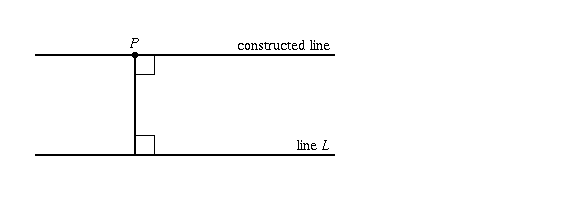Eventually it was discovered that the parallel postulate is logically independent of the other postulates, and you get a perfectly consistent system even if you assume that parallel postulate is false. This means that it is possible to assign meanings to the terms "point" and "line" in such a way that they satisfy the first four postulates but not the parallel postulate. These are called non-Euclidean geometries. Projective geometry is not really a typical non-Euclidean geometry, but it can still be treated as such.

In this axiomatic approach, projective geometry means any collection of things called "points" and things called "lines" that obey the same first four basic properties that points and lines in a familiar flat plane do, but which, instead of the parallel postulate, satisfy the following opposite property instead:

The projective axiom: Any two lines intersect (in exactly one point).
(Depending on how one words the other axioms, they may need some slight modification too).

Using only this statement, together with the other basic axioms of geometry, one can prove theorems about projective geometry. Many of them are the same as ordinary geometry; the big difference is that there is no such thing as a pair of parallel, non-intersecting lines in projective geometry.

One interesting fact is worth mentioning: in projective geometry, points and lines are completely interchangeable! That is, any statement about points and lines would still be true even if you replaced all occurrences of the word "point" with the word "line", and vice versa. For instance, the basic axiom that "for any two points, there is a unique line that intersects both those points", when turned around, becomes "for any two lines, there is a unique point that intersects (i.e., lies on) both those lines", which is the property described above. There is a complete duality between points and lines in projective geometry.

Now, if this approach were all there was to projective geometry, it would be little more than an intellectual curiosity. All it means is that it logically consistent for there to be concepts called "points" and "lines" that satisfy the axioms of geometry with the projective axiom in place of the parallel postulate. It says nothing about whether such concepts would be interesting, relevant, or have any relation whatsoever to the normal concepts of lines and planes in Euclidean geometry.

However, there are other approaches that reveal the connection:

## 2. Euclidean Geometry Plus A "Line At Infinity"

Another way to approach projective geometry is to define it as follows:

Take each line of ordinary Euclidean geometry and add to it one extra object called a "point at infinity". Do this in such a way that the same extra object is added to parallel lines (so that the extended lines now intersect), while different extra objects are added to non-parallel lines (so that the extended lines don't intersect more than once).

In other words:

• To each line l of Euclidean geometry, associate some other object f(l), in such a way that f(l) = f(l') if and only if l and l' are parallel.

[There are lots of ways to do this. For example, you could let f(l) be the slope of l (a real number, or the symbol " infinity " if l is vertical). Alternatively, you could let f(l) be the counterclockwise angle from some fixed reference line to l. The precise method you use is unimportant.]

• The points of projective space are the points in Euclidean geometry together with these additional objects f(l) (which are called the points at infinity).

• The lines of projective space are lines l in Euclidean space together with the extra object f(l) attached. In addition, the collection of all the extra objects together is also called a line in projective space (called the line at infinity).

This definition satisfies all the axioms of projective geometry. For example, here's a proof that any two of these "lines" L and L' intersect in exactly one "point":

If one of L and L' (say, L) is the line at infinity and the other (L') is not, then they intersect at exactly one point because by definition L' contains exactly one point at infinity.

If neither L nor L' is the line at infinity, then each of them consists of an ordinary Euclidean line together with one point at infinity. That is, we can write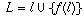andwhere l and l' are Euclidean lines.

If l and l' intersect at a point p then f(l) does not equal f(l') (since f(l) only equals f(l') when l and l' are parallel), so p is the one and only intersection point of L and L'.

If l and l' do not intersect, then f(l) = f(l') (since l and l' are parallel), so again L and L' have exactly one intersection point.

This view of projective geometry makes it relatively easy to answer questions of concurrence and collinearity.

For example, what does a collection of concurrent lines in projective space look like? It is one of three things:

1. a collection of the projective extensions of lines that were concurrent in Euclidean space, or
2. a collection of the projective extensions of lines that were parallel in Euclidean space (but are now concurrent at a point at infinity), or
3. case 2 above together with the line at infinity.
As for collinearity: points that are collinear in Euclidean space are still collinear in projective space. Also, the points at infinity are all collinear in projective space. A point p at infinity is only collinear with a collection of finite points if p = f(l) where l is the Euclidean line through the finite points.

Although this view of projective geometry helps answer your question, it's still a little artificial, with all this talk of just "adding extra objects at infinity". There are two other, much more natural, ways of looking at it.

## 3. Lines In Space

Projective geometry can be thought of as the collection of all lines through the origin in three-dimensional space. That is, each point of projective geometry is actually a line through the origin in three-dimensional space. The distance between two points can be thought of as the angle between the corresponding lines. A line in projective geometry is really a family of lines through the origin in three-dimensional space.

To see how this ties in with the previous view of projective geometry, let P be a horizontal plane in space that does not pass through the origin. As can be seen in the picture below, every line through the origin passes through exactly one point on P, except for the horizontal lines.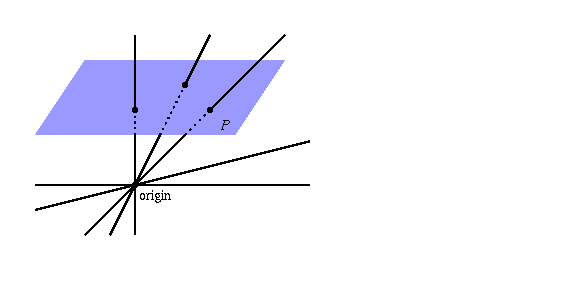So there is a one-to-one correspondence between the points on the ordinary plane P, and some of the points in projective space (namely, all non-horizontal lines through the origin in 3-d space). The remaining points in projective space are horizontal lines through the origin in 3-d space; these are the "points at infinity".

Now think about a line l in P. This corresponds to a family of lines through the origin in 3-d space, as shown. As you move to infinity on the line l, the corresponding lines through the origin actually converge to a horizontal line parallel to l, so this limiting line should be included in the family.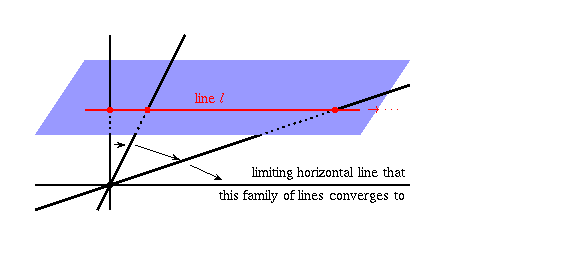You can see this explicitly if you look at the direction vectors of the lines. Suppose that the plane P is at height 1 above the origin in 3-d space, and you have a line l given by y = mx + b on P. Then a typical point p on l has 3-d coordinates (x, mx+b, 1). This means that (x, mx+b, 1) is a direction vector for the line through the origin that passes through p. The unit direction vector is obtained by dividing this vector by its length, to getIt is an exercise in limits to show that this converges to (1,m,0) as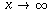; in other words, this family of lines converges to a horizontal line through the origin with slope m. Notice that the intercept b does not appear in the limit: parallel lines l, l' in P (ones with the same m and different b) correspond to families of lines through the origin in 3-d space which converge to the same horizontal line through the origin.

What all this means is that, in projective space, the "line" corresponding to l is actually a family of lines through the origin consisting of: (1) the lines that pass through l, and (2) the limiting horizontal line. Using the language in which a line through the origin in 3-d space is called a "point" in projective space, and a horizontal one is called a "point at infinity" in projective space: the line in projective space corresponding to the Euclidean line l on P consists of (1) the points in projective space that correspond to the points on l, and (2) the point at infinity in projective space that is parallel to l.

This is the same as what we saw before: lines in projective space consist of lines in Euclidean space with an added point at infinity. The difference is that now there's a definite geometric interpretation of the points at infinity. They're not just artificially added fabrications; rather, they're horizontal lines through the origin in 3-space. The remaining, non-horizontal lines through the origin in 3-space are in one-to-one correspondence with the points in a standard Euclidan plane P.

## 4. A Non-Orientable Surface

There's yet another way to understand projective geometry: it is the geometry of curves on a rather weird surface. This surface cannot be embedded in the standard 3-dimensional world we live in; you'd need to live in 4 dimensions to visualize it completely. Nevertheless, it has the following fairly easy interpretation: take a sphere (spheres in mathematics are hollow surfaces, not solid balls), and think of gluing together all pairs of antipodal points so that they become the same point. (Antipodal points are those which are on the most opposite part of the sphere from each other. For instance, if the centre of the sphere is at the origin, then the antipodal point of (x,y,z) is (-x,-y,-z).)

Another way to think of it is to just take the top hemisphere, and then "seal it up" into a closed surface by gluing each point on the equator to its opposite point.

Now, if you put a sphere with its centre at the origin in 3-d space, then every line through the origin passes through exactly 2 antipodal points in the sphere (and therefore exactly one point in our surface, after those two antipodal points get glued onto the same spot). So, there is a one-to-one correspondence between points in projective space (lines through the origin in 3-d space) and points on this weird surface.

What is a "straight line" on a surface like a sphere or our glued surface? It's not really straight, obviously, but it can still be defined as giving the shortest distance between two points. On a sphere, the shortest path between two points is an arc that is part of a "great circle" on the sphere (one whose centre is the centre of the sphere). So, the "straight lines" on a sphere are the great circles.

The points on the top hemisphere of the sphere (excluding the equator) correspond to the points in a standard Euclidean plane as shown in the picture below. The points on the equator are the "points at infinity". Intersecting lines in the standard Euclidean plane are great circles that intersect as shown on the sphere:Parallel lines in the standard Euclidean plane are great circles that intersect only at the equator, as shown below: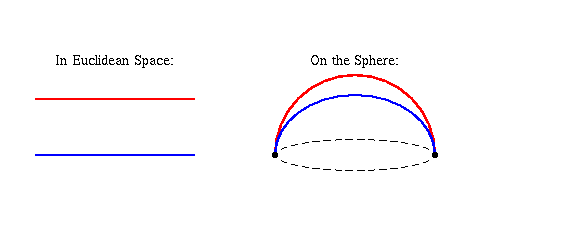(note that although these great circles intersect in two points, they become the same point after antipodal points get glued together so it's still true that in projective space, any two lines intersect in exactly one point).

In summary, then, projective geometry can be thought of as the study of points and "lines" (great circles) on a surface obtained by gluing the equator of a hemisphere to itself. It's very similar to the study of points and "lines" (great circles) on a sphere, except that on a sphere every pair of lines intersects in exactly two (antipodal) points instead of one.

[ Submit Your Own Question ] [ Create a Discussion Topic ]

This part of the site maintained by (No Current Maintainers)
Last updated: April 19, 1999
Original Web Site Creator / Mathematical Content Developer: Philip Spencer
Current Network Coordinator and Contact Person: Joel Chan - mathnet@math.toronto.edu

Navigation Panel: